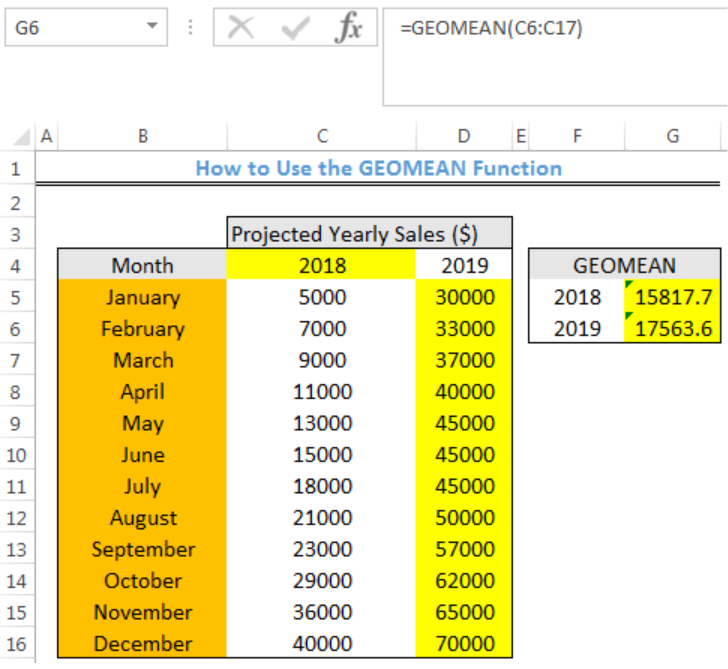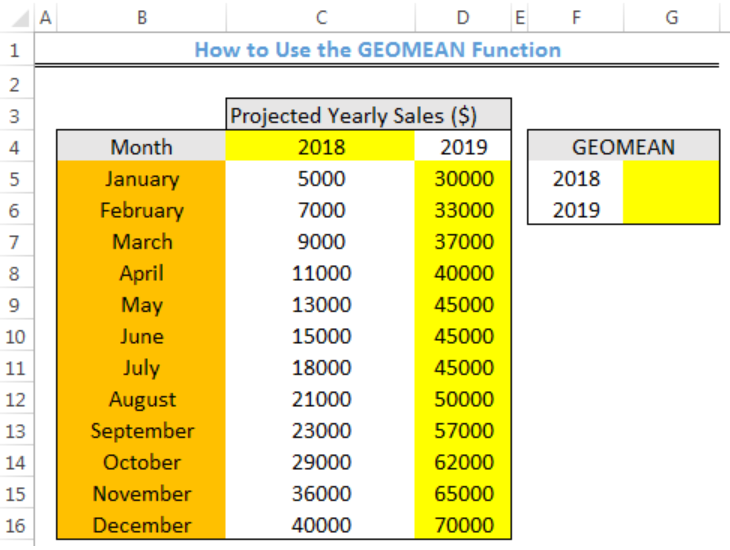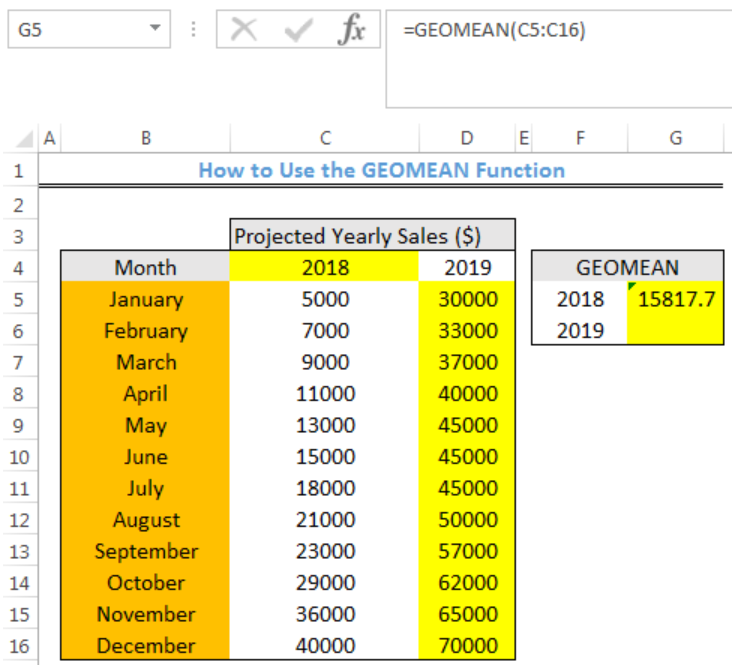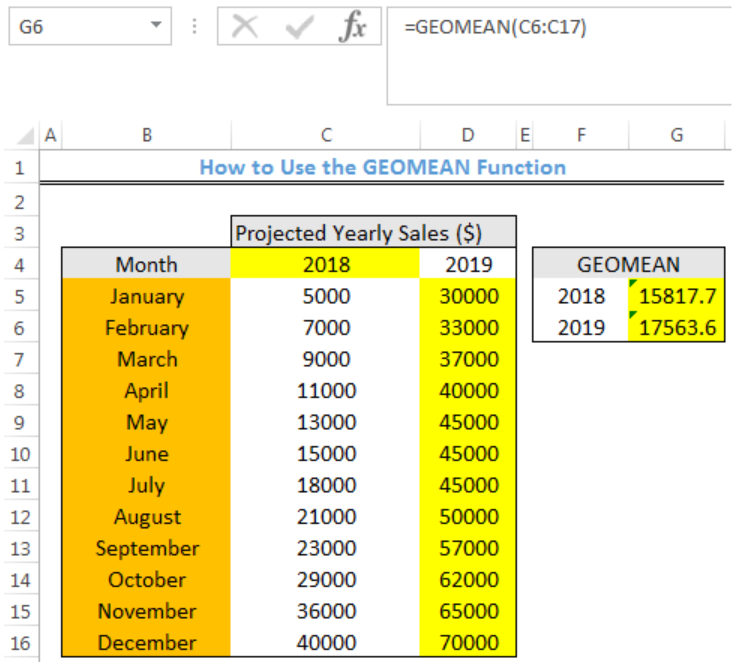Get instant live expert help with Excel or Google Sheets“My Excelchat expert helped me in less than 20 minutes, saving me what would have been 5 hours of work!”

#### Post your problem and you’ll get expert help in seconds.

Your message must be at least 40 characters
Our professional experts are available now. Your privacy is guaranteed.

# How to Calculate the Geometric Mean in Excel

We can use the GEOMEAN function to calculate the average rate per period for a set of values that is compounded over multiple periods. The geometric mean is the average rate of return of a set of values calculated with the products of the terms. The steps below will walk through the process.Figure 1- Final result of the GEOMEAN function

## Syntax

`=GEOMEAN(number1, [number2], ...)`

• number1: This is a number or cell reference that refers to the first value
• number2 [optional]: This is a number or cell reference that refers to the second value

## Formula

`=GEOMEAN(C5:C16)`

## Setting up the Data

We will calculate the geometric mean for the sales of year the 2018 and 2019 in figure 2.

• The months will be entered into Column B
• Column C and D contain the Sales for 2018 and 2019 respectively
• The Geometric mean sales for each year will be returned in Column GFigure 2 – Setting up the Data

## Geometric Mean Sales for 2018 and 2019

• We will click on Cell G5
• We will insert the formula below into Cell G5
`=GEOMEAN(C5:C16)`
• We will press the enter keyFigure 3- Geometric Mean Sales for 2018

• We will click on Cell G5 again
• We will double click on the fill handle tool which is the small plus sign you see at the bottom right of Cell G5. Select and drag down to copy the formula to Cell G6.Figure 4- Geometric Mean Sales for 2018 and 2019

## Explanation

`=GEOMEAN(30000,33000) returns 31464.3`

The GEOMEAN function calculates the geometric mean of a set of numbers by returning the nth root of n numbers. Hence, the Geomean of 30000 and 33000 is calculated as:

`=(30000*33000)^(1/2)`

`=31463.3`

The arithmetic mean would be `(30000 + 33000)/2 = ``31500`.

## Note

• Any empty cell and cell with logical values within the range are ignored by the GEOMEAN Function
• The GEOMEAN Function can treat up to 255 different arguments. These arguments include numbers, cell references, ranges, arrays, and constants.

## Instant Connection to an Expert through our Excelchat Service

Most of the time, the problem you will need to solve will be more complex than a simple application of a formula or function. If you want to save hours of research and frustration, try our live Excelchat service! Our Excel Experts are available 24/7 to answer any Excel question you may have. We guarantee a connection within 30 seconds and a customized solution within 20 minutes.

Are you still looking for help with the Average function? View our comprehensive round-up of Average function tutorials here.